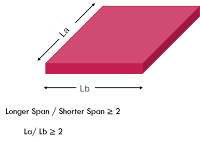# DIFFERENCE BETWEEN ONE WAY SLAB AND TWO WAY SLAB - CIVIL ENGINEERING NOTES

Slab is one of the most basic component of a structure and there are many types of slab that has been used in a building for many reasons. A slab is basically a two-dimensional bending member that is designed for bending, shear, and torsion at the corners. It is one of the most efficient members of a structure. It is constructed to provide flat surfaces, usually horizontal, in building floors, roofs, bridges, and other types of structures. Basically, the slab is divided into two main types, one-way slab and two-way slab

## One-Way Slab:Definition: According to ACI codes, the slab will be called as one-way slab if the ratio of longer span to shorter span is equal to or greater than 2. Let take an example we have a slab of 6m x 3m, here 6m is the longer span which is La and 3m is the shorter span which is Lb so 6m divide by 3m is equal to 2 which is equal to 2 so according to the ACI codes, we can say that this particular slab is a one-way slab.

### Bending moment:

One-way slab, the slab bends only in one direction which is in the direction of its shorter
span.

### Supports:

The one-way slab is supported by the beams on two opposite sides. Examples for one-way slab are veranda.

In one way slabs, loads are transferred from slabs to beams along one direction.

### How the reinforcement is used:

One-way slab bends in one direction so to overcome this bending we provide main steel which is also called cranked bars on the shorter side of the slab.

### Slab thickness:

While designing one-way slab we do not provide any distribution bars so the amount of steel is less and because of that the depth of one-way slab increases and due to that, the thickness of the one-way slab is more as compared to the two-way slab.

### Uses:

If the span length is up to 3.6m then it is economical to use a one-way slab instead of a two-way slab.

## Two-Way Slab:

### Definition:According to per ACI codes, In the case of the two-way slab, the ratio of longer span to shorter span is less than 2. Suppose we have a slab of 5m x 3m. in this case, 5m is the longer span and 3m is the shorter span so 5m divide by 3m and is equal to 1.5m which is less than 2. So we can say that this is a two-way slab.

### Bending moment:

Two-way slab the slab bends in both directions that are in the direction of longer span and shorter span.

### Supports:

Two-way the slab is supported by beams on all four sides. Examples for two-way slabs are used in the construction of multi-stories building which normally made of frame structures.

In the case of the two-way slab, loads are transferred to beams in both directions.

### How the reinforcement is used:

In case of the two-way slab, the slab bends in both directions so to overcome that bending we provide main steel, also called the cranked bar on both sides of the slab.

### Slab thickness:

when we design two-way slab we provide distribution bars which means the more steel will be used and because of that we provide less depth, so the thickness of two-way the slab is less as compared to the one-way slab.

### Uses:

Two-way slab is economical for panels size up to 6mx6m.

Watch the video for a better understanding